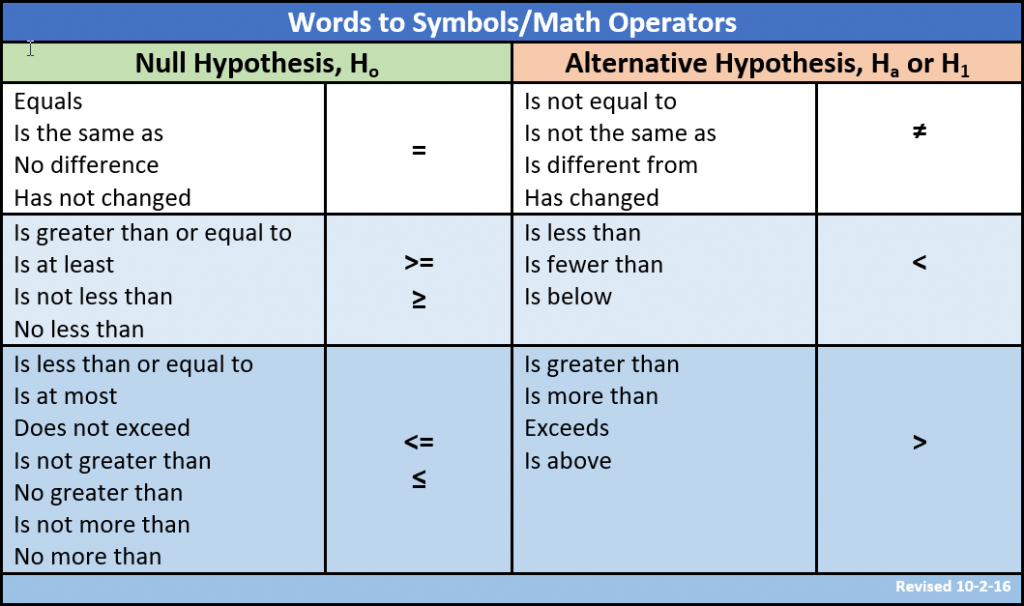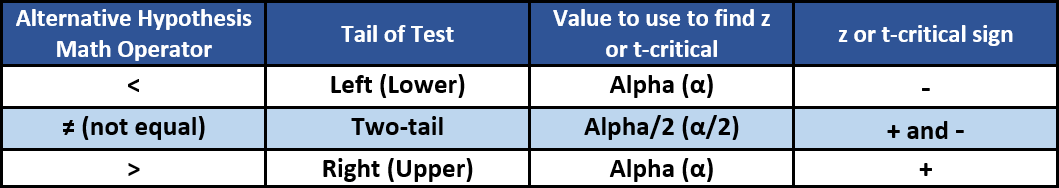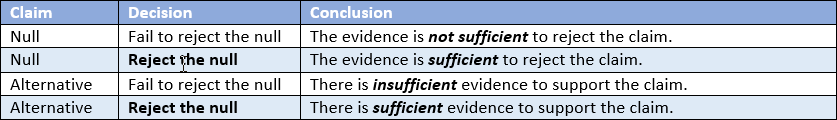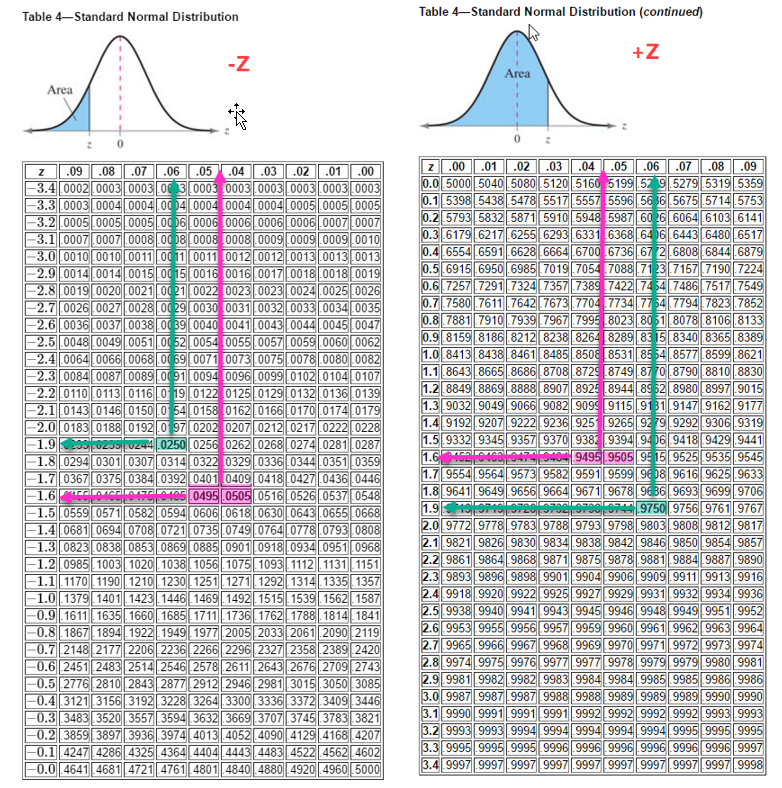# Thoughts on Section 7.4 for Mia

Mia,

In rereading your email, I am seeing several problems you say you are having with the section on proportions.

1. Identify the test
When you are working with one or two proportions, you always use a Z-test. Knowing that simplifies the calculation of the critical value – we are always looking for values of Z.
2. Find the critical values of Z
To find the critical value(s) and associated rejection areas, you need to know alpha and you need to know how many tails and the direction of tails in the test.

Alpha is almost always given in a problem statement. If you do run into a rare occasion where alpha is not given, using 0.05 is usually very safe.

Identifying the tails/direction of the test depends upon accurately stating the null and alternative hypotheses. To do that, I think this table helps tremendously:

Table 1Read the problem statement carefully looking for the key words in the table. For example, “the instructor believes that more than 70% of his students met the state standard.” The words “more than” clue you to the > symbol which is in the alternative hypothesis column. Thus the alternative is Ha: p > 0.70. The null and alternative are always complementary. I set up the table so that complementary nulls and alternative are in the same colored row. Staying in the blue row, we find the ≤ symbol in the null hypothesis side of the table. This means the null is Ho: p ≤ 0.70.

The math operator in the alternative always determines the tails of the test. A < points to the left and tells us the test is a left-tailed (sometimes called lower-tailed) test.

Table 2Here are some graphs showing the three types of tests:The first is a left tail test with alpha=0.05 and gives a Z-critical of -1.645. The rejection area (in red) includes all Z-values less than or equal to -1.645.

The second is a two-tailed test, still with alpha = 0.05. We put alpha/2 or 0.025 in each tail which leaves 0.95 between the tails (red area). The critical values of z are -1.96 and +1.96. The rejection areas (in white this time) include all z-values less than or equal to -1.96 and all z-values greater than or equal to +1.96.

The third is a right tail test with alpha=0.05 and gives a Z-critical of +1.645. The rejection area (in red) includes all Z-values greater than or equal to +1.645.

To read examples of finding the critical value of Z from tables, go to the end of this post.

To see how to use the TI 84 calculator to find a critical value of Z given alpha or alpha/2, go here (Note: move to the 5-minute mark to actually get to use of the TI InvNorm function.)

Recall that the area under the normal curve represents probability and equals 1. Probability always must be greater than or equal to 0 and less than or equal to 1, i.e. 0 ≤ P ≤ 1.

Thus the rejection area on a left tail test represents a probability of 0.05 or a 5% chance the standardized test statistic Z falls in it and a 95% chance it does not. Similarly, there is a 5% chance the test statistic falls in the rejection area for the right-tail test.

But notice that a test statistic, say Z=1.7, that just falls in the rejection area of a right tail test would not fall within the rejection area for a two-tailed test with the same alpha, i.e. 1.7 is greater than 1.645 but is not greater than 1.96. That means the effect size (the standardized test statistic) in a two-tailed test must have a greater absolute value to be determined to be statistically significant and to indicate the null should be rejected. We talk about the reason one might or might not choose a two-tailed test instead of a one-tailed test in a later post.

1. Identify the Claim

Next we must identify the claim so that we might draw a conclusion from the result of our hypothesis test. Some authors subscribe to the ‘burden of proof’ concept when setting the claim and state the claim must be the alternative if you want to prove it to be true. This idea is important for serious researchers, but often confuses folks learning statistics for the first time. I subscribe to the idea that the claim can be either the null or the alternative hypothesis. Recall, we cannot prove the null to be true even though we assume it is true. All we can do is to ‘fail to reject’ it, which I think is sufficient for most purposes.

To decide which alternative is the claim, all you have to do is to look at the math operators in the two hypotheses, which we determined using Table 1 above. In the example I gave, “the instructor believes that more than 70% of his students met the state standard,” the words “more than” clue us to the > symbol which has to be in the alternative hypothesis. This is the claim and thus the alternative hypothesis is the claim.

If the wording in the example had been, “the instructor believes that no more than 70% of his students met the state standard,” the words “no more than” clue you to the symbol which is in the null hypothesis column. This would make the null the claim.

See how easy this approach is?

1. Calculate the test statistic and/or the p-value.
The p-value is not the same as the critical value. The p-value is a probability, not a z-value. The critical value is a z-value, not a probability.
1. Decide to reject the null or fail to reject the null.
• If your test statistic falls into a rejection region, you reject the null. Otherwise, you fail to reject the null.
• If your p-value is less than alpha, you reject the null. Otherwise, you fail to reject the null.
1. Write your conclusion. Note: this section applies to all forms of hypothesis tests.
• If your claim is the null and you fail to reject the null, you can conclude the evidence is not sufficient to reject the claim.
• If your claim is the null and you reject the null, you can conclude the evidence is sufficient to reject the claim.
• If your claim is the alternative and you fail to reject the null, you can conclude there is insufficient evidence to support the claim.
• If your claim is the alternative and you reject the null, you can conclude there is sufficient evidence to support the claim.

Notice the pattern? When you have sufficient evidence (a large enough effect size) to support the alternative, you reject the null. When you do not have sufficient evidence (the effect size is not large enough) to support the alternative, you fail to reject the null. The following table highlights this pattern.

Table 3Finding Critical Values of Z from the Standard Normal Tables

To find the left critical value as shown in the sketch below, we need to enter the appropriate value of the area in the Z tables. Remember, the standard normal tables always give the total area under the curve from left infinity to the value of interest.

In our example above, we have an alpha of 0.05. For a left tail test, we put all of alpha in the tail and thus we should enter the negative Z table with a value of 0.05. Looking through the table, we find 0.0495 and 0.0505 (pink) but no 0.0500. So we interpolate between the two and look left on that row to find a Z of -1.6 and then up to find a value of 0.045. Combining those together, we get a value of -1.645 for a left critival value of Z.

For our two-tailed test, we enter with alpha/2 or 0.025 to find the negative value of Z-critical. This time we do find exactly 0.0250 (green)so we do not need to interpolate. Following the row to -1.9 and the column to 0.06, we combine them to find the left critical value of Z of -1.96. Now we should remember that because the normal curve is symetrical, the right value of Z for alpha/2 would be +1.96. But let’s actually use the positive Z table to find it.This time we need to remember the table has the area to the left of Z, but we need the area to the right of Z. Using the complement rule, we subtract alpha/2 from 1 (i.e. 1 -.025) to get 0.975. And we again find that value (green) in the positive Z table. Looking left and up we find 1.9 and .06 which combine to 1.96 for our Z-critical value for the two-tailed test.

For the right tail test, we perform a similar operation, 1 – alpha or 1 – 0.05 = 0.95 for the value we need to enter the positive Z table. Again, we do not find that exact value (pink) and again must interpolate to find +1.6 and 0.045, which combine to our critical value of +1.645 for our right tail test.